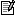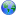Last update March 7, 2012

#Scientific and Mathematical Libraries in DThis list only includes libraries which are in a usable state. All libraries use a liberal license (eg, BSD, Academic Free) unless otherwise stated.Scientific and Mathematical Libraries in Dtango.math (D1.x)MultiArraydstats (D2.x)blip (D1.x)OpenmeshOnpLylaSciDScrapple/backmathScrapple/unitsBindingsPlotting librariesDplotPLplot libraryPlot2KillWishlistAbandoned / Proof-of-Concept libraries

##tango.math (D1.x)Version D1.x D2.x Library Tango• Mathematical Special Functions (80-bit)
• Cumulative distribution functions (80-bit).
• Random number generation for several elementary probability distributions.
• BigInt.
• 1-D root finding and minimisation via bracketing.

##MultiArrayVersion D1.x D2.x Library Phobos• Wrappers: wrappers for various high-performance linear algebra libraries: BLAS, LAPACK, UMFPACK, SuperLU, TAUCS, and ARPACK.
• Murray: an N-dimensional array class that emulates a multidimensional-array. BLAS/LAPACK are used to implement maxtrix-vector and matrix-matrix multiplication, linear solver, and a least squares solver.
• DFlat: a Vector/Matrix library implementing various storage formats of dense and sparse matrices (dense: strided,banded,packed; sparse:compressed row, compressed column, triplet). This additionally includes a simple, unified interface to the linear solvers in the LAPACK, UMFPACK, SuperLU and TAUCS libraries.

##dstats (D2.x)Version D1.x D2.x Library PhobosLicense Mostly Boost a couple borrowed modules are under BSD
• D2 port of parts of tango.math
• correlation: Pearson, Spearman, and Kendall correlation, and covariance.
• Entropy, mutual information, conditional mutual information.
• Random number generation for several elementary probability distributions.
• Sorting algorithms with some added features that are useful for non-parametric statistics calculations.
• Summary statistics such as: mean, median, standard deviation, skewness, kurtosis.
• Hypothesis testing, such as T-tests, Wilcoxon tests, Chi-square and Kolmogorov-Smirnov tests.
• Linear regression with statistical inference capabilities.

##blip (D1.x)Version D1.x D2.x Library Tango• SMP parallelization
• serialization
• random testing framework
• N dimensional arrays with a nice interface to several lapack functions.

##OpenmeshVersion D1.x D2.x Library Phobos• OpenMesh is a library for manipulating polygonal meshes.

##OnpVersion D1.x D2.x Library Phobos Phoboshttp://smp.if.uj.edu.pl/~baryluk/d/onpd/onp/ddoc/general.html

##LylaVersion D1.x D2.x LibraryDsourceProject:lyla Template-class library for matrices and vectors, dense and sparse.

##SciDVersion D1.x D2.x Library PhobosSciD is a collection of numerical routines and bindings written in and for the D programming language. Currently, the collection is rather small but expanding.

For the time being, SciD simply aims to be a freely usable repository of numerical algorithms. The long-term goal is to ultimately produce a full-fledged, general-purpose scientific library � la the GNU Scientific Library or SciPy?.

Components:

• scid.calculus: Numerical integration (quadrature) and differentiation.
• scid.constants: Fundamental constants of mathematics and Nature.
• scid.exception: Functions and classes used by SciD? for exception handling, also useful for client code. (Similar to std.exception.)
• scid.functions: Mathematical special functions.
• scid.linalg: Linear algebra functions, i.e. user-friendly interfaces to LAPACK.
• scid.matrix: Contains the MatrixView? type, which is a LAPACK-compatible matrix view of ordinary D arrays, as well as some utility functions.
• scid.nonlinear: Methods for nonlinear equation solving.
• scid.types: Various useful types.
• scid.util: Various odds and ends that don't fit anywhere else.

##Scrapple/backmathVersion D1.x D2.x Library Phobos##Scrapple/unitsVersion D1.x D2.x Library Phobos#Plotting libraries##DplotVersion D1.x D2.x Library Phobos• A wrapper for gnuplot

##PLplot libraryVersion D1.x D2.x Library##Plot2KillVersion D1.x D2.x Library Phobos#Wishlist• A graphing package
• Basic 2-D x-y plots, linear/log/user-defined(eg, statistical distribution), support for infinity and nan. -> see PLplot library above.

#Abandoned / Proof-of-Concept librariesThese libraries contain functionality which may be useful for further library development.

| | | | | | | | | |

(date of last change: March 7, 2012 20:37 )